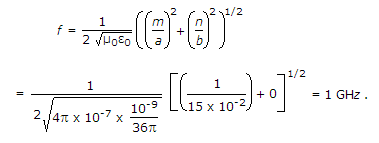# Electronics and Communication Engineering - Exam Questions Papers - Discussion

4.

A rectangular waveguide, in dominant TE mode, has dimensions 10 cm x 15 cm. The cut off frequency is

 [A]. 10 GHz [B]. 1 GHz [C]. 15 GHz [D]. 25 GHz

Explanation:

For TE10 modeVasundhara said: (Aug 16, 2014) In general dimensions of rectangle are represented as l:b. So a = 10cm, but here they have considered a = 15cm. Can any one help me to understand this?

 Alok said: (Apr 13, 2015) Answer will be 1.5 GHz. For TE mode we will consider a = 10 cm and f= (3*10^10) / (2*a (cm)).

 Santhosh said: (Apr 5, 2016) Please explain clearly. I can't understand.

 Gopal said: (Sep 19, 2016) @Alok. I agree with your answer. For Dominant mode TE10. Cutoff frequency f = c/2a. Put all the value and we get our answer.

 Gauri said: (Jan 20, 2018) Yes, answer should be 1.5 GHz.

 Rajesh said: (Feb 24, 2018) Dominant mode is TE10 only when dimensions are a>b but here in the question he gave a

 Navya Rekha said: (Mar 23, 2018) @Gauri. Here a is wider band& b is narrow band means a=15,b=10. So,f=3*10^8 m/2*15 cm, 3*10^8/0.3=1ghz.

 Thenmozhi said: (Nov 20, 2018) @Gopal. What is "c" here?